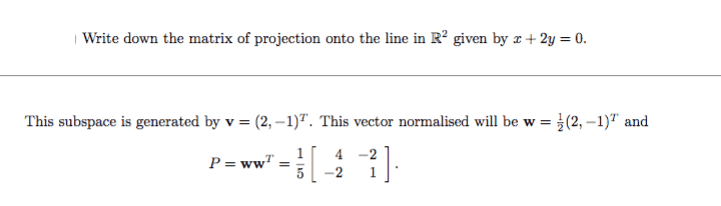# Projection matrix

• UOAMCBURGER

## Homework Statement[/B]

## The Attempt at a Solution

The solution is obviously given, but I don't really understand what is done there. What method is being used? so I can understand, because i see how they attained v, but then that vector normalised is not correct is it?

#### Attachments

You (and we) need the Relevant Equations filled out.

What is a Projection Matrix? What kinds of properties does it have that we can take for granted?

You (and we) need the Relevant Equations filled out.

What is a Projection Matrix? What kinds of properties does it have that we can take for granted?
Don't worry, I learned how to solve it now. since you can find the projection matrix pretty easily then you just plug in the values from the given vector, here W (unit vector of v) the squares cancel the square roots and you're left with that matrix in solution P. But i was wondering how do you add a matrix into the text?

Last edited by a moderator:
•SammyS and UOAMCBURGER